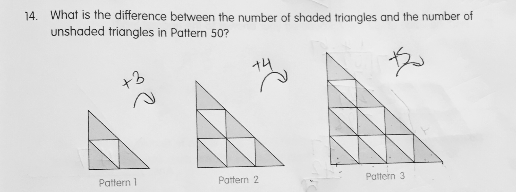# QuestionAnyone help me with attached question

Thanks

Thank you so much

0 Replies 0 Likes

The formula for the answer is just pattern number + 1.

0 Replies 1 Like

Difference between the number of shaded triangles and the number of unshaded triangles in Pattern :
1 ——- 1 + 1 = 2
2 ——- 2 + 1 = 3
3 ——- 3 + 1 = 4

50  ——- 50 + 1 = 51

Ans : 51.

0 Replies 1 Like

Difference between the number of shaded triangles and the number of unshaded triangles in Pattern :
1 ——- 1 + 1 = 2
2 ——- 2 + 1 = 3
3 ——- 3 + 1 = 4

50  ——- 50 + 1 = 51

Ans : 51.

0 Replies 1 Like

Notice the number of unshaded triangles in a pattern is the same as that of the shaded triangles in the previous pattern.

For example in pattern 3, the number of unshaded triangles is 1+2+3=6;

the number of shaded triangles in pattern 2 is also 6.

From observation the number of shaded triangles in pattern 50 would be the sum of (1+2+3+…+51);

the number of unshaded triangles in pattern 50 would be (1+2+3+…+50).

The difference is 51.

0 Replies 1 Like